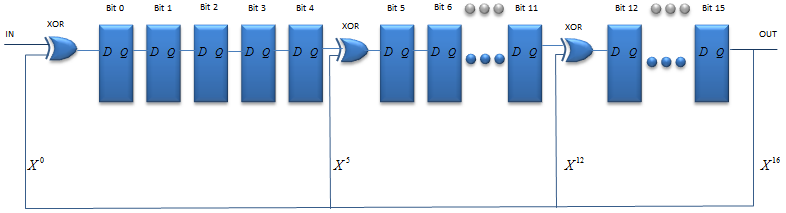Electronics - Shift Register                                                  Home : www.sharetechnote.com Shift Register is a special type of register that takes in a bit sequence and shift it by one bit at every rising edge of the clock towards output pin. Overall diagram would look as follows. (There can be more pins (e.g, Clear pin) in real implentation). This illustration shows an example where we implement it using D FlipFlop, but you can implement it using other types of Flip Flop (e.g, JK FlipFlop) as well.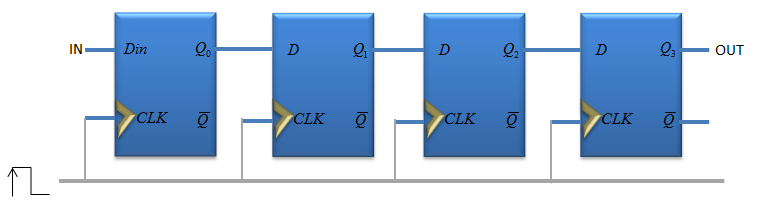One example of timing diagram for this is as follows. (If you are not familiar with D FlipFlop operation mechanism, I would suggest you to read D Flip Flop page first.)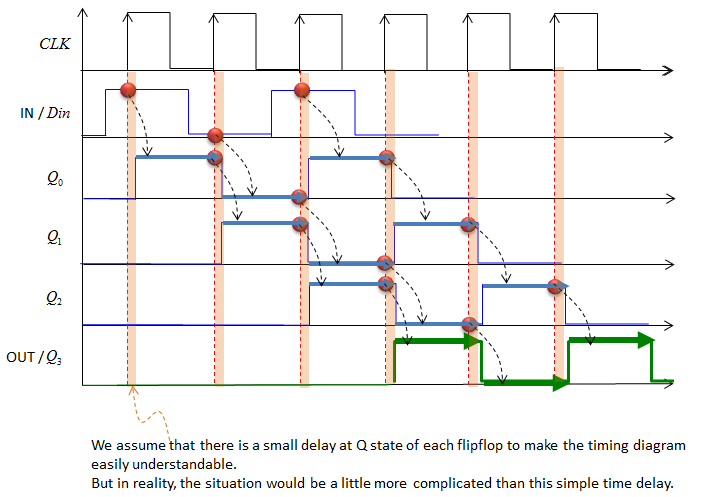Applications     Serial to Parallel Converter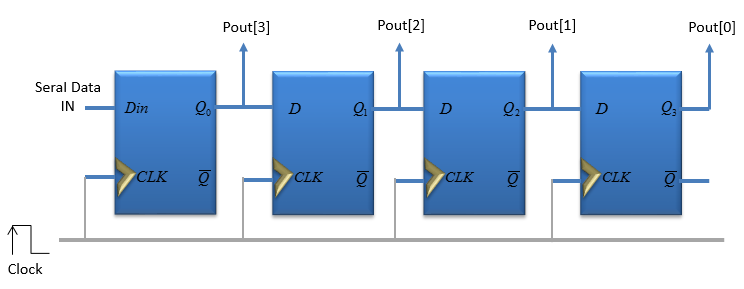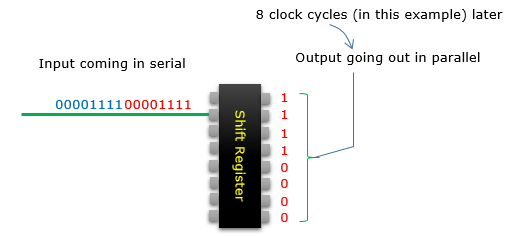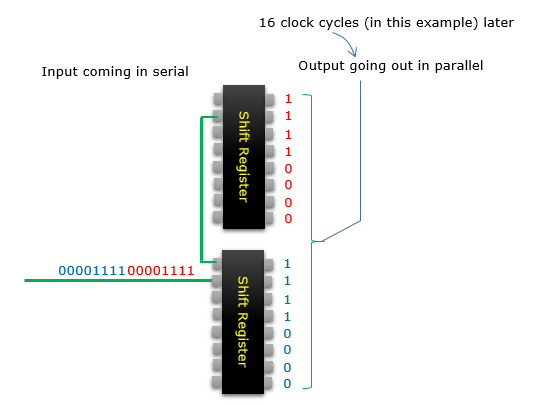CRC Calculator   One of the most common example (especially in digital communication) for the application of Shift Register is CRC calculator. For example, if you want to calculate the following CRC polynomial. (If you are not familiar with CRC concept and CRC polynomial, see the CRC page)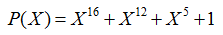You can implement a shift register as follows. ( For simplicity, I didn't show CLOCK and other pins)